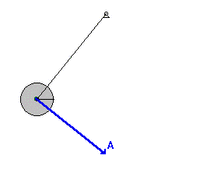# Mechanical energy facts for kids

Kids Encyclopedia FactsA swinging pendulum with the velocity vector (green) and acceleration vector (blue). The magnitude of the velocity vector, the speed, of the pendulum is greatest in the vertical position and the pendulum is farthest from Earth in its extreme positions.

In physics, mechanical energy describes the potential energy and kinetic energy present in the components of a mechanical system.

When a given amount of mechanical energy is transferred (such as when throwing a ball, lifting a box, crushing a soda can, or stirring a beverage) it is said that this amount of mechanical work has been done. Both mechanical energy and mechanical work are measured in the same units as energy in general. It is usually said that a component of a system has a certain amount of "mechanical energy" (i.e. it is a state function), whereas "mechanical work" describes the amount of mechanical energy a component has gained or lost.

The conservation of mechanical energy is a principle which states that under certain conditions, the total mechanical energy of a system is constant. This rule does not hold when mechanical energy is converted to other forms, such as chemical, nuclear, or electromagnetic. However, the principle of general conservation of energy is so far an unbroken rule of physics - as far as we know, energy cannot be created or destroyed, only changed in form.

## Images for kidsMechanical energy Facts for Kids. Kiddle Encyclopedia.